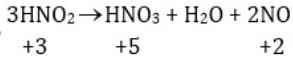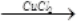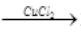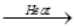Courses

# Short & Long Answer Question (Part-1) - The p block elements Class 12 Notes | EduRev

## Chemistry Class 12

Created by: Mohit Rajpoot

## Class 12 : Short & Long Answer Question (Part-1) - The p block elements Class 12 Notes | EduRev

The document Short & Long Answer Question (Part-1) - The p block elements Class 12 Notes | EduRev is a part of the Class 12 Course Chemistry Class 12.
All you need of Class 12 at this link: Class 12

Q.1 Write the elements of group 15?

Ans. The elements of group 15 are Nitrogen (N), Phosphorous (P) , Arsenic (As) , Antimony (Sb) and Bismuth (Bi).
Q.2  Write chemical name & formulae of

a) Chile saltpetre

b) Indian saltpetre

Ans. (a) Chile saltpetre – Sodium nitrate – NaNO3

(b) Indian saltpetre – Potasium nitrate –KNO3
Q.3 What is special about the valence configuration of Group 15?

Ans. The valence configuration of 15 group is ns2np3 the s-orbital is completely filled and p-orbital is half filled . This half filled orbital gives extra stability to elements of this group.
Q.4  The atomic radii increases considerably from N to P but very little increase is observed from As to Bi. why?

Ans. There is a considerable increase in size from N to P as expected but due to the presence of completely filled d- orbitals which have very poor shielding effects, the increases in size is very little from As to Bi.
Q.5 Give reason for the following- the first ionization enthalpy of 15th group elements is higher than 16th group elements ?

Ans. Due to extra stability of half filled configuration, the first Ionisation enthalpy of 15th

group elements is higher than 16th group configuration ns2np3
Q.6 How does metallic character vary down the 15 group & why?

Ans. The metallic character increases down the group due to decrease in ionization enthalpy and increase in size of atom.
Q.7 What are the common oxidation states of this group?
Ans. The common oxidation states of the group are -3, +3 & +5.
Q.8 What is the maximum covalence shown by N?

Ans. Nitrogen shows a maximum covalence of +4 because only four orbitals, one S and three P- orbitals are available for bonding in Nitrogen.
Q.9 Bi (v) is a stronger oxidizing agent than Bi(III). Why?

Ans. Bi is more stable in +3 oxidation state in comparison to +5 due to inert pair effect

therefore Bi (v) has a strong tendency to act as oxidizing agent.
Q.10 Give an example showing disproportionation of oxidation state of nitrogen?
Ans.Here Nitrogen is getting oxidized to a higher oxidation state as well as reduced to a lower oxidation state.

Q.11 Complete and balance -
(i) (NH4)2SO4 + 2NaOH →
(ii)  2FeCl3(aq) + 3NH4OH(aq)→
(iii) AgCl(s) + 2NH3(aq)→
(iv) NaNO3 + H2SO4
(v) 3NO2(g) + H2O(l)→
(vi) Cu + 4HNO3(conc)→

(vii) 4Zn + 10HNO3 (dil)→
(viii) [Fe(H2O)6]2+NO→
(ix) I2 10HNO3
(x) Ss + 48 HNO3(conc)→
Ans. (i) (NH4)2 SO4 + 2 NaOH → 2NH3 + 2H2O + Na2SO4
(ii) 2FeCl3(aq) + 3NH4OH(aq) → Fe2O3.xH2O(s) + 3NH4Cl(aq)
(iii) AgCl(s) + 2NH3(aq) → [Ag(NH3]2] Cl(aq)
(iv) NaNO3 + H2SO4 → NaHSO4 + HNO3
(v) 3NO2(g) + H2O(l)→ 2HNO3(aq) + NO(g)
(vi) Cu + 4HNO3(conc) → Cu(NO3)2 + 5H2O +N2O
(vii) 4Zn + 10HNO3(dil) → 4Zn(NO3)2 + 5H2O + N2O
(viii) [Fe(H2O)6]2+ + NO → [Fe(H2O)5(NO)]2+ + H2O
(ix) I2 + 10HNO3 → 2HIO3 + 10 NO2 + 4H2O
(x) S8+48HNO3(conc) → 8H2SO4 + 48NO2 + 16H2O
Q.12 What are the optimum conditions for maximum yield of ammonia?
Ans. The optimum conditions for the production of ammonia are - 200*105 Pa or 200 atm pressure, 700K temperature , and presence of catalyst such as iron oxide with K2O and Al2O3 as promoters.
Q.13 Ammonia is a Lewis base. Why?

Ans. Due to the presence of lone pairs on nitrogen atom of ammonia, it can donate electron pair and acts as a lewis base.
Q.14 Ammonia has higher boiling and melting points than expected. Why?

Ans. In solid and liquid states, ammonia molecules are associated by inter-molecular

hydrogen bonding. There fore ammonia has higher boiling and melting points.
Q.15  Give reasons for the following:-
a)Halogens have smallest atomic radii in their periods

b) The negative electron gain enthalpy of fluorine is less than that of chlorine.

c) All halogens are coloured.

d) The only possible oxidation state of fluorine -1.

e) Fluorine forms only one oxoacid.

f) The stability of hydrides follows the order HF>HCl>HBr>HI.

Ans.  (a) Due to maximum effective nuclear charge, halogens have smallest atomic radii.

(b) Due to small size of fluorine atom, there are strong inter electronic repulsions in the

small 2p orbital of fluorine and thus incoming electron does not experience much attraction and fluorine has less negative electron gain enthalpy than that of chlorine.

(c) Halogens absorb radiation in visible region which results in oxidation of electrons to

higher energy level by absorbing different quanta of radiation, they show different colours.

(d) Since fluorine is most electronegative element and is short of only one electron for

completing octet, it shows the only oxidation state of -1.

(e) Due to small size and high electro-negativity, Fluorine forms only one hypohalous acid.
(f) As the size of element increases down the group, the bond dissociation enthalpy for HX bond decreases making the bond weaker and weaker therefore the order of thermal stability is HI < HBr < HCl < HF.
Q.16 Complete and balance-
(i) 2F(g) + 2H2O(l)→
(ii) 4NaCl + MnO2 + 4H2SO4
(iii) 4HCl + O2(iv) C10H16 + 8Cl2
(v) 6NaOH + 3Cl2
Ans. (i) 2F2(g) + 2H2O(l) → 4H+(aq) + 4F- + O2(g)
(ii) 4NaCl + MnO2 + 4H2SO4 → MnCl2 + 4NaHSO4 + 2H2O + Cl2
(iii) 4HCl + O22Cl2 + 2H2O
(iv) C10H16 + 8Cl2 → 16HCL + 10C
(v) 6NaOH + 3Cl2  → 5NaCl + NaCiO3 + 3H2O

Q.17 Chlorine water on standing loses its yellow colour. Why?
Ans. On standing chlorine water forms HCl and Hypochlorous acid (HOCl) due to which it loses its colour. Cl2 + H2O → HCL + HOCI
Q.18 . Explain the bleaching action of chlorine?
Ans. The bleaching action of chlorine is due to its tendency to give nascent oxygen so that the substance gets oxidized.  Cl2 + H2O ---> 2HCl +[O]
Coloured substances + [O] → colourless substance.
Q.19 Write two uses of chlorine?

Ans. Chlorine is used for

a) Bleaching wood pulp, cotton and textiles

b) Manufacturing dyes, drugs, refrigerants etc.

c) Sterilizing drinking water.
Q.20 Give reasons for the following?
(a) Nitrogen does not show catenation.
(b) PCl5 exists but NCl5 does not.

(c) The stability of Hydrides follows the order-
NH3>PH3>Ar5H3>SbH3

(d) PH3 is a weaker base than NH3
(e) Molecular nitrogen is chemically inert.
Ans. (a) Nitrogen being small in size has high electron density. Due to strong inter electronic repulsions, N-N single bond is weak & nitrogen does not undergo catenation.
(b) Due to absence of d-orbitals, nitrogen cannot expand its oxidation state to +5 and NCl5 does not exist whereas in P due to presence of empty 3d orbital +5 oxidation state is attained.
(c) As we move down the group 15, atomic radii increases making the bond of element with Hydrogen weaker this decreases the stability of hydrides of heavier elements. Therefore the order of stability is.
NH3>PH3>AsH3>SbH3
(d) As Phosphorous atom is larger than N- atom, the lone pair of electrons is distributed over a large surface area of P-atom than N-atom. Therefore the tendency of P to donate the lone pair of electrons is less.

(e) Molecular nitrogen (N2) is inert because N N bond energy is very high due to small size of N- atom and presence of multiple bond.
Q.21 Complete and balance-
(a) NH4Cl(aq) + NaNO2(aq)→
(b) Ba(N3)2
(c) 3Mg + N2
(d) N2 + O2(e) 2NaN3
Ans. (a) NH4Cl(aq) + NaNO2(aq) → N2(g) + 2H2O(l) NaCl(aq)
(b) Ba(N3)2 → Ba + 3N2
(c) 3Mg + N2Mg3N2
(d) N2 + O22NO(g)
(e) 2NaN3  → 2Na+3N2
Q.22 What are the two isotopes of nitrogen? Write two uses of dinitrogen.
Ans. The two isotopes of nitrogen are 14N and 15N
Q.23 Write the characteristics of pure Ozone?
Ans. Pure ozone is pale blue gas, dark blue liquid and violet black solid
Q.24 At what concentration ozone is harmful?
Ans. If the concentration of ozone increases above 100 ppm, breathing becomes uncomfortable resulting in headache and nausea and it becomes harmful.
Q.25 Explain the oxidizing action of ozone?
Ans. Ozone has a very strong tendency to liberate nascent oxygen according to the equation:- O3 O2+O
Therefore it acts as a strong oxidizing agent.
Q.26 How is ozone estimated quantitatively?
Ans. When ozone reacts with an excess of potassium iodide solution buffered with a borate buffer (PH = 9.2) iodine is liberated. This iodine can be titrated against a standard solution of sodium thiosulphate to estimate the amount of ozone.
2I-(aq)+H2O + O3 → 2OH- (aq) + I2(s) + O2(g)
Q.27 Complete and balance:-
(i) PbS(s) + 4O3(g)→
(ii) NO(g) + O3(g) →
(iii) 4FeS2(s) + 11O2(g)→
(iv) 2NaOH + SO2
(v) 2Fe3+ + SO2 +2H2O →
(vi) Cu + 2H2SO4(conc) →
Ans. (i) PbS(s) + 4O3(g) → PbSO4(s) +4O2(g)
(ii) NO(g) + O3(g) → NO2(g) + O2(g)
(iii) 4FeS2(s) + 11O2(g) → Na2SO3 + H2O
(iv) 2NaOH + SO2 → Na2SO3 + H2O
(v) 2Fe3+ + SO2 + 2H→ O2Fe2+ + SO2-+ 4H+
(vi) Cu + 2H2SO4(conc) → CuSO4 + SO2 + 2H2O
Q.28 Give a test to detect the presence of SO2 gas?
Ans. When SO2 (g) is passed through a violet coloured acidified potassium permanganate solution, it gets decolourised.
5SO2 + 2MnO-4 + 2H2O → 5SO2-4 + 4H+ + 2Mn2+
Q.29 Which aerosols and oxides deplete ozone layer

Ans. Freon used in aerosol sprays deplete the ozone layer. Nitrogen oxides emitted from the exhaust systems of supersonic jet aeroplanes deplete the ozone layer.
Q.30 Write the members of 16 Group.
Ans. The elements of group 16 are: Oxygen(O), Sulphur (S), Selenium (Se), Tellurium (Te) and Polonium (Po)
Q.31 Give the general electronic configuration of 16 Group.
Ans. The general electronic configuration of 16 group is ns2np4 .
Q.32 Discuss the geometry of SF4.
Ans.
In SF4 the hybridisation of sulphur is sp3d. The structure is trigonal bi-pyramidal in which one of the equatorial positions is occupied by a one pair of electrons. The geometry is called see-saw geometry.
Q.33 Enlist some uses of dioxygen.
Ans. Oxygen is used-
(a) In oxyacetylene welding.
(b) In manufacture of steel.
(c) In hospitals and mountaineering as oxygen cylinders.
Q.34 Write different isotopes of oxygen.
Ans. The isotopes of oxygen are  16O, 17O, 18O.
Q.35 Which of the following does not react with oxygen directly? Zn, Ti, Pt, Fe
Ans. Pt is a noble metal and does not react very easily. All other elements, Zn, Ti, Fe, are quite reactive. Hence, oxygen does not react with platinum (Pt) directly.
Q.36 Why is ICl more reactive than I2 ?
Ans. ICl is more reactive than I2 because I-Cl bond in ICl is weaker than I-I bond in I2 .
Q.37 Balance the following equation:
XeF6 + H2O → XeO2F2 + HF
Ans. Balanced equation: XeF6 + H2O → XeO2F2 + 4HF
Q.38 How is ammonia manufactured industrially?
Ans. Ammonia is prepared on a large-scale by the Haber's process.
N2(g) + 3H2(g) → 2NH3(g)
Q.39 Why does R3P = O exist but R3N = O does not (R = alkyl group)?
Ans. N(unlike P) lacks the d-orbital. This restricts nitrogen to expand its coordination number beyond four. Hence,R3N = O  does not exist.
Q.40 . Explain why fluorine forms only one oxoacid, HOF.
Ans.
Fluorine forms only one oxoacid i.e., HOF because of its high electronegativity and small size.
Q.41 Which one of the following does not exist?
(i) XeOF4 (ii) NeF2 (iii) XeF2 (iv) XeF6
Ans. NeFdoes not exist.

Offer running on EduRev: Apply code STAYHOME200 to get INR 200 off on our premium plan EduRev Infinity!

,

,

,

,

,

,

,

,

,

,

,

,

,

,

,

,

,

,

,

,

,

;